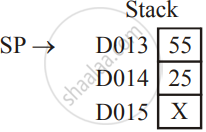HSC Science (Computer Science) 12th Board ExamMaharashtra State Board
Share

# Explain the Function of Following Instructions of Intel 8085 : Push B - HSC Science (Computer Science) 12th Board Exam - Computer Science 2

#### Question

Explain the function of following instructions of Intel 8085 :

PUSH B

#### Solution

PUSH B [PUSH REGISTER PAIR ON STACK]

Formal: [[SP] - 1] ← [rh]

[[SP] - 2] ← [rl]

[[SP] ← [SP] - 2

Bytes: 1 byte

Flags: None

Comment :

1) The contents of the higher order register of register pair rp are moved to the memory location, whose address is one less than the content of stack points.

2) The contents of the low order register or register pair rp are moved to the memory location whose address is two less than the content of stack pointer.

3) The stack pointer is determined by two. rp may be any one of the B (B & C), D (D & E), H (H & L)

Example:

Let [SP] = D015 H, [B] = 25 H and [C] = 55 H

Instruction: PUSH B

After execution: [D014] = 25 H

[D013] = 55 H

and [SP] = D013 HIs there an error in this question or solution?

#### APPEARS IN

2017-2018 (March) (with solutions)
Question 4.1.1 | 3.00 marks
Solution Explain the Function of Following Instructions of Intel 8085 : Push B Concept: Instruction Set and Programming of 8085.
S